## Agenda

• High-level graphics
• Custom graphics
• Layered graphics in `ggplot2`

## Functions for graphics

• The functions `hist()`, `boxplot()`, `plot()`, `points()`, `lines()`, `text()`, `mtext()`, `axis()`, etc. form a suite that plot graphs and add features to the graph
• `par()` can be used to set or query graphical parameters

## Univariate data: Histogram

```x = state.x77[ , 2]                   # 50 average state incomes in 1977
hist(x)```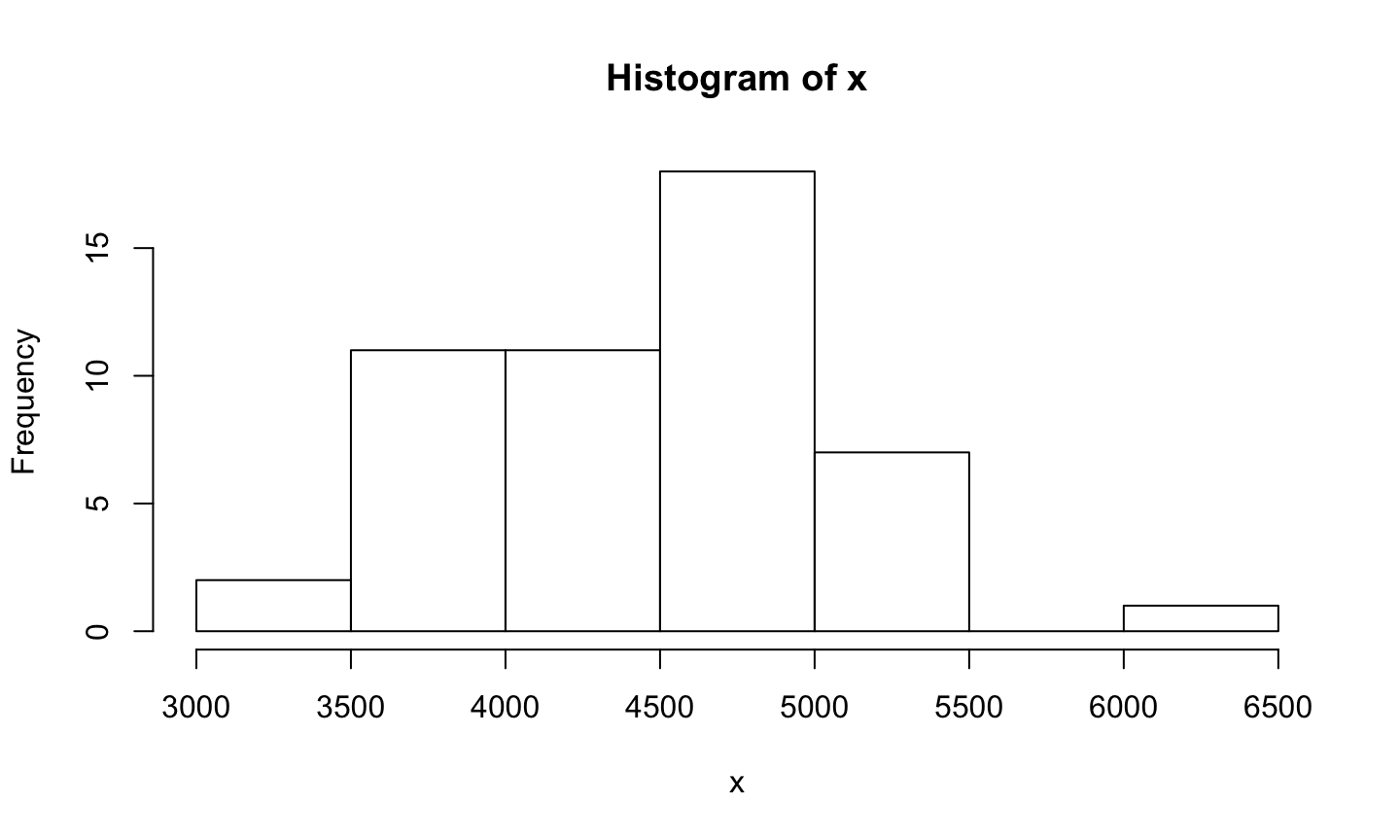## Univariate data: Histogram

`hist(x, breaks = 8, xlab="Income", main="Histogram of State Income in 1977")`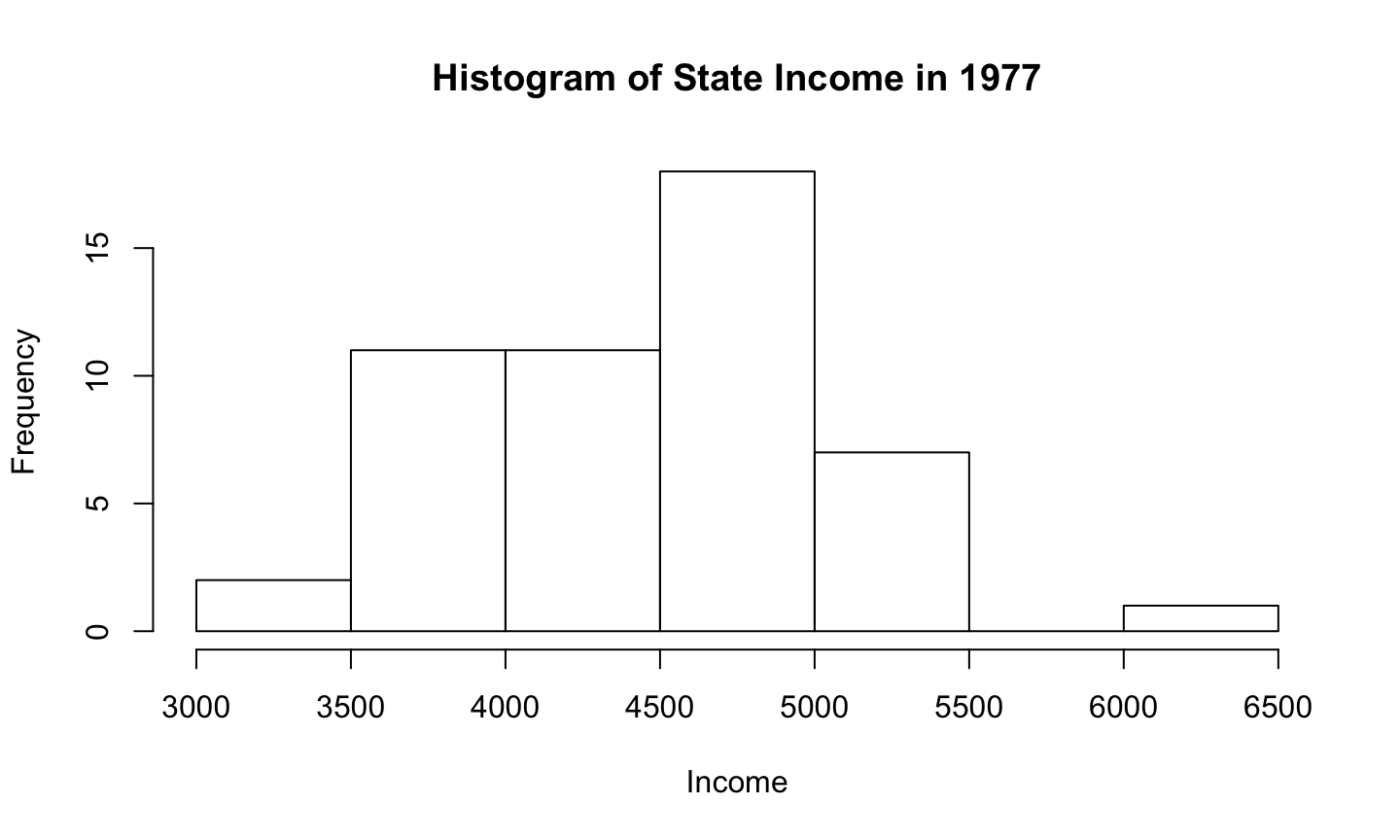## Univariate data: Histogram

```y = quakes\$depth                      # 1000 earthquake depths
hist(y, seq(0, 700, by = 70), xlab="Earthquake Depth", main="Histogram of Earthquake Depths")```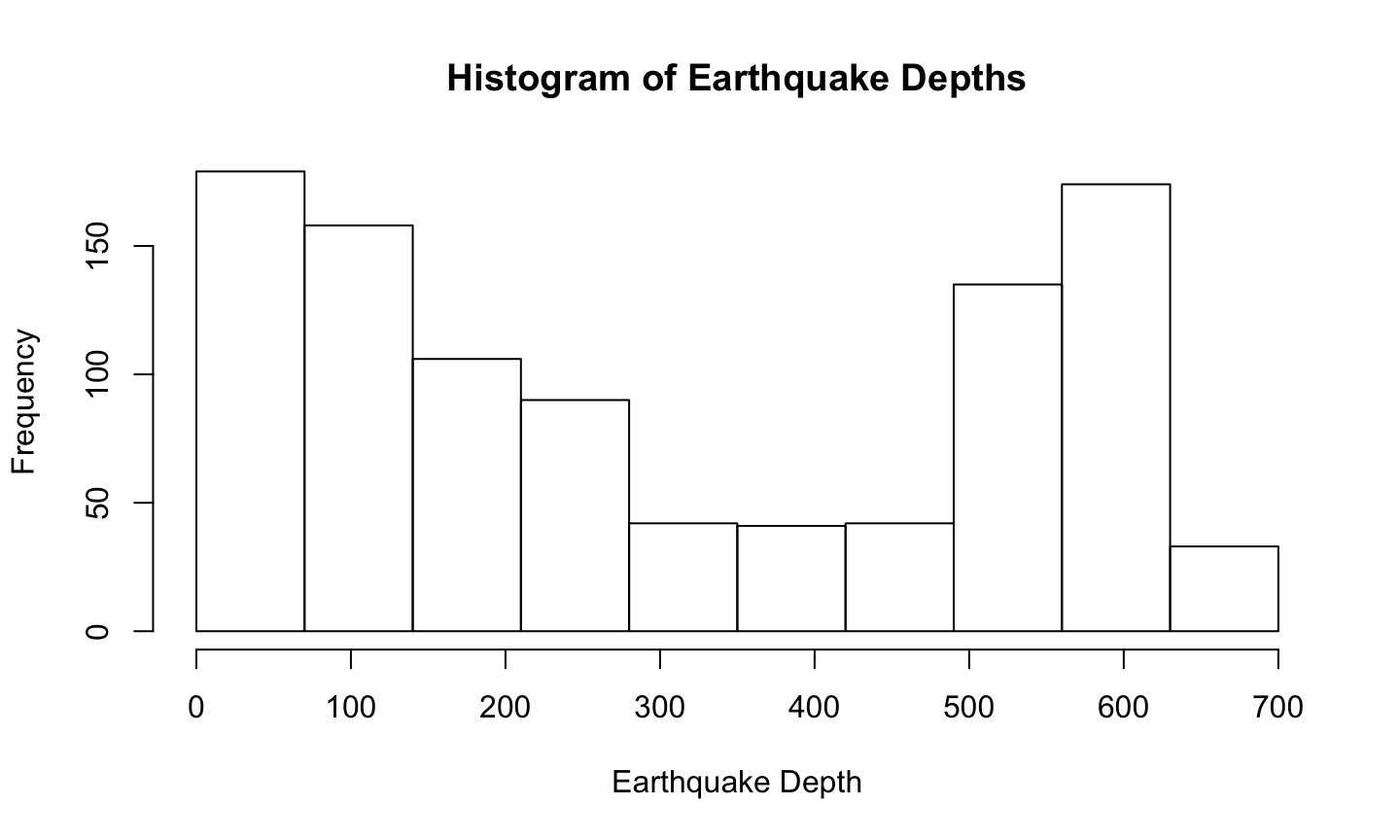## Empirical CDF

Function `ecdf()` provides data for empirical cdf

`plot.ecdf(x)`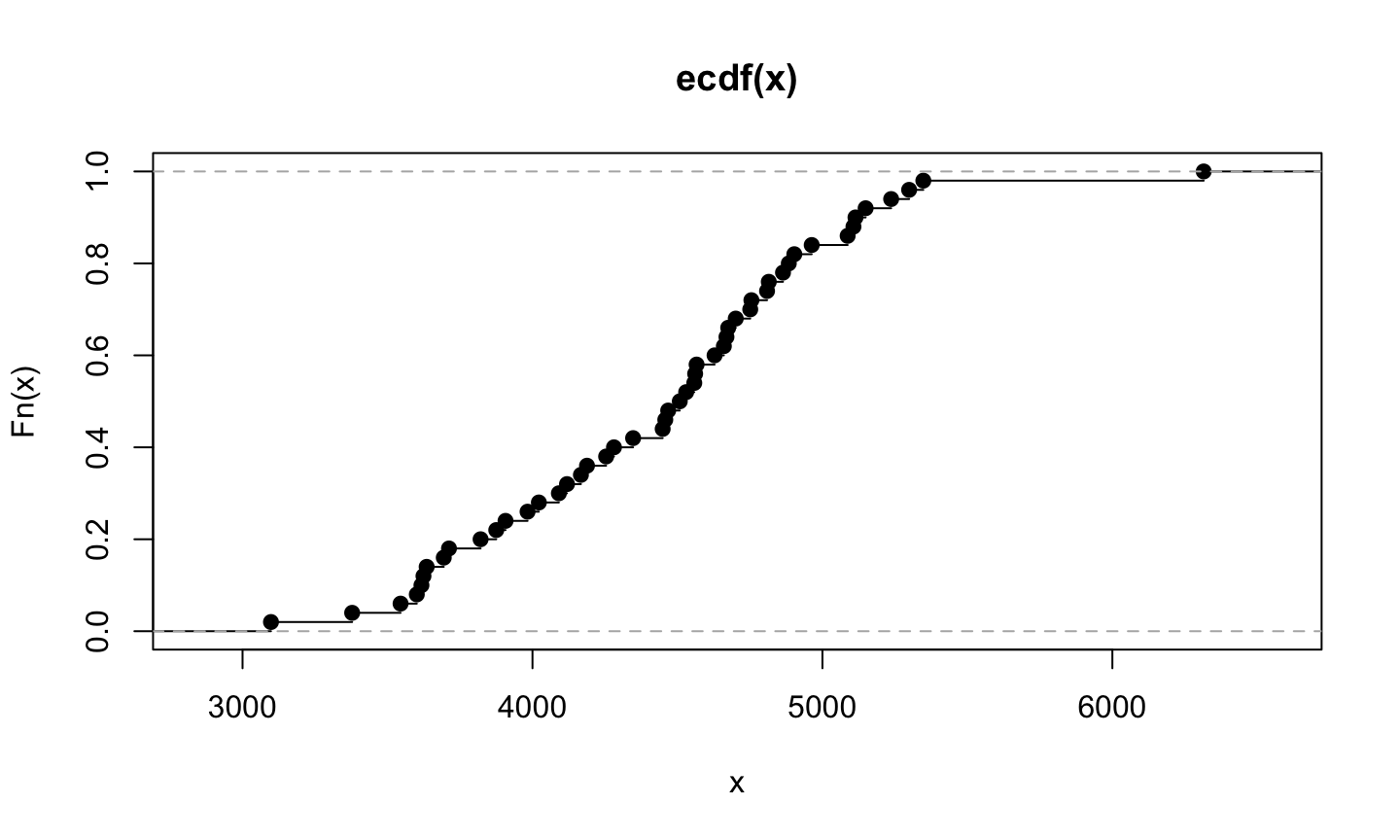## Empirical CDF

Can add vertical lines and remove dots

`plot.ecdf(x, verticals = T, pch = "", xlab="Income", main="ECDF of State Income in 1977")`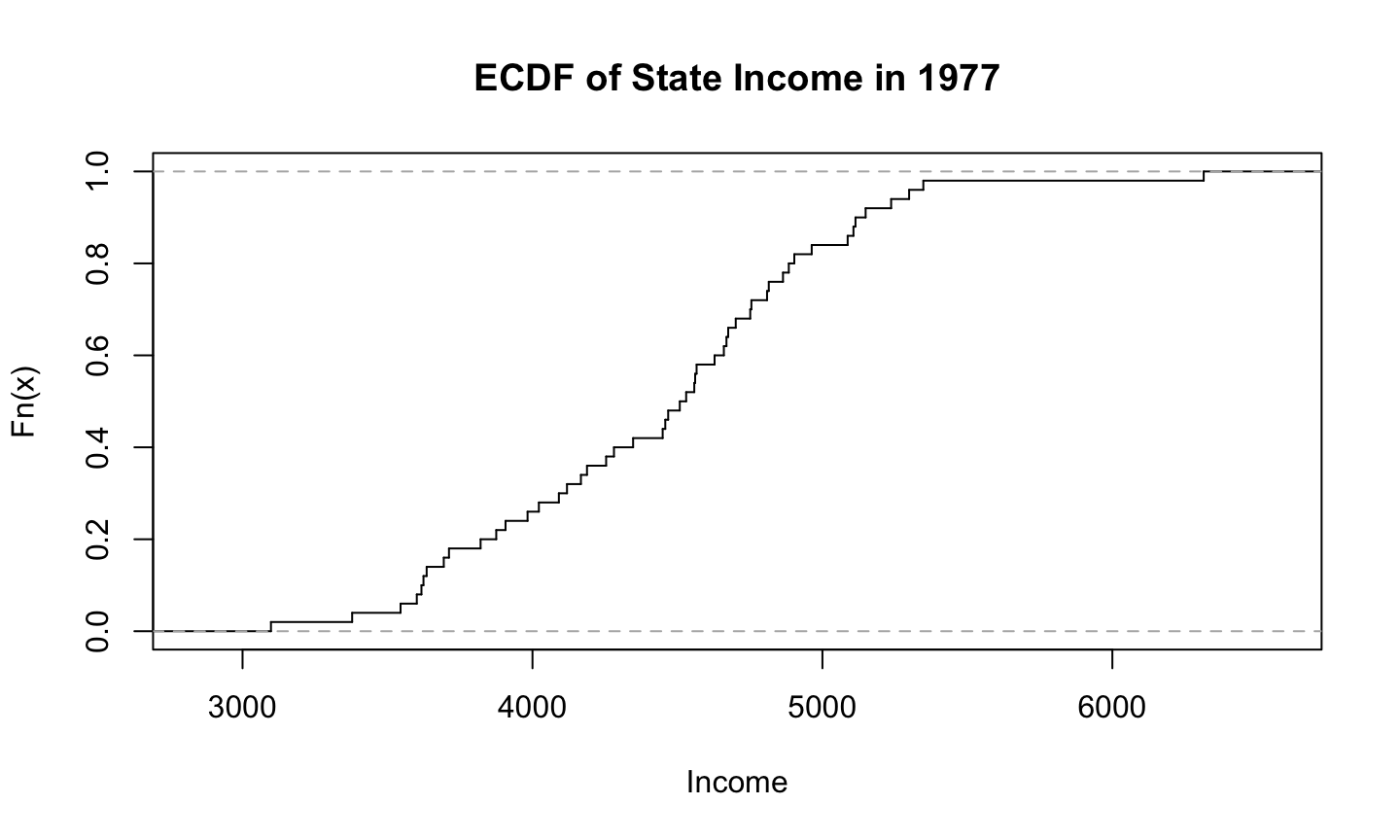## Empirical CDF

```plot.ecdf(y, verticals = T, pch = "", xlab="Earthquake Depth",
main="ECDF of Earthquake Depths")```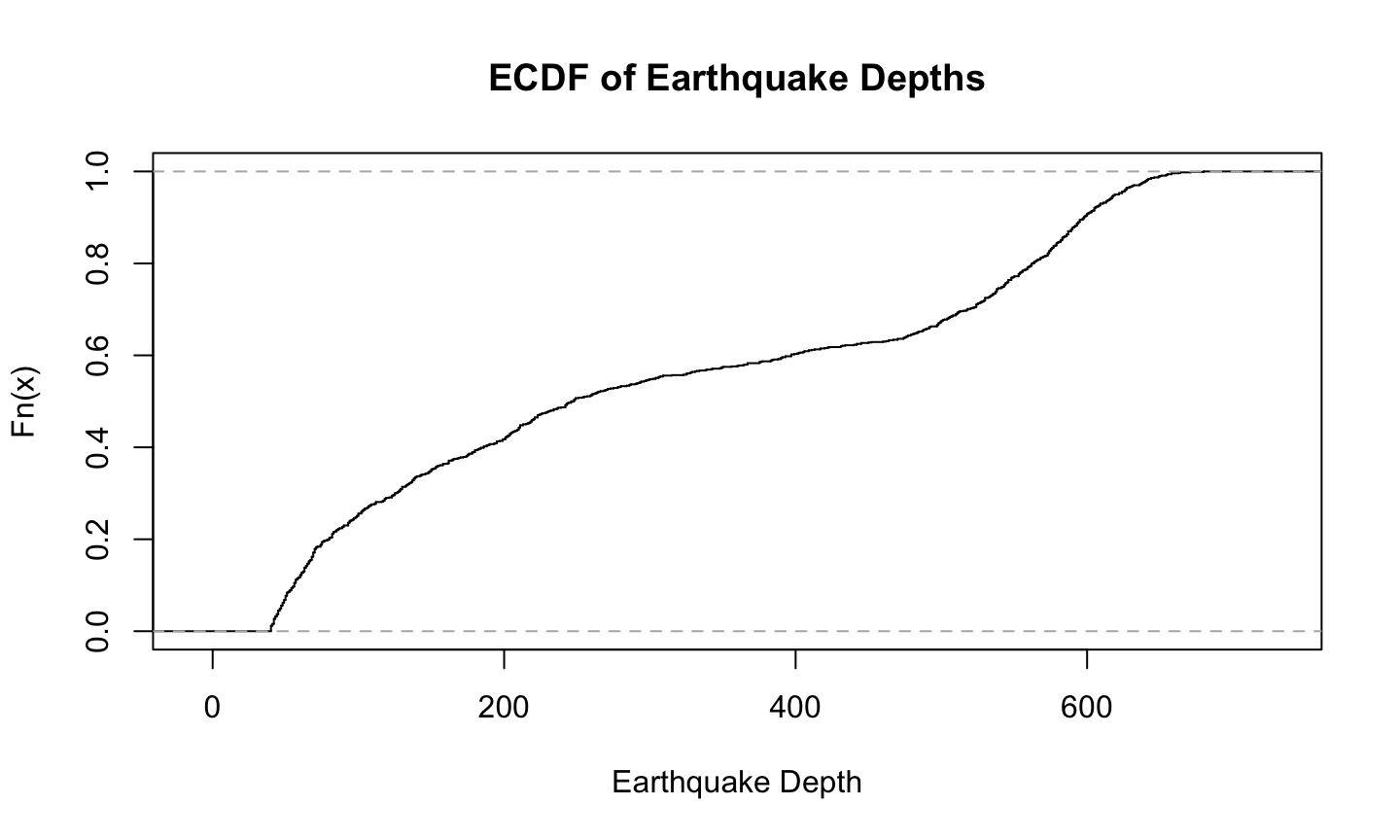## `qqnorm()` and `qqplot()`

• `qqnorm() plots the quantiles of a data set against the quantiles of a Normal distribution
• `qqplot() plots the quantiles of a first data set against the quantiles of a second data set

## `qqnorm()` and `qqplot()`

```qqnorm(x)                             # qq plot for the earthquake depths
qqline(x, col = "red")                # red reference line```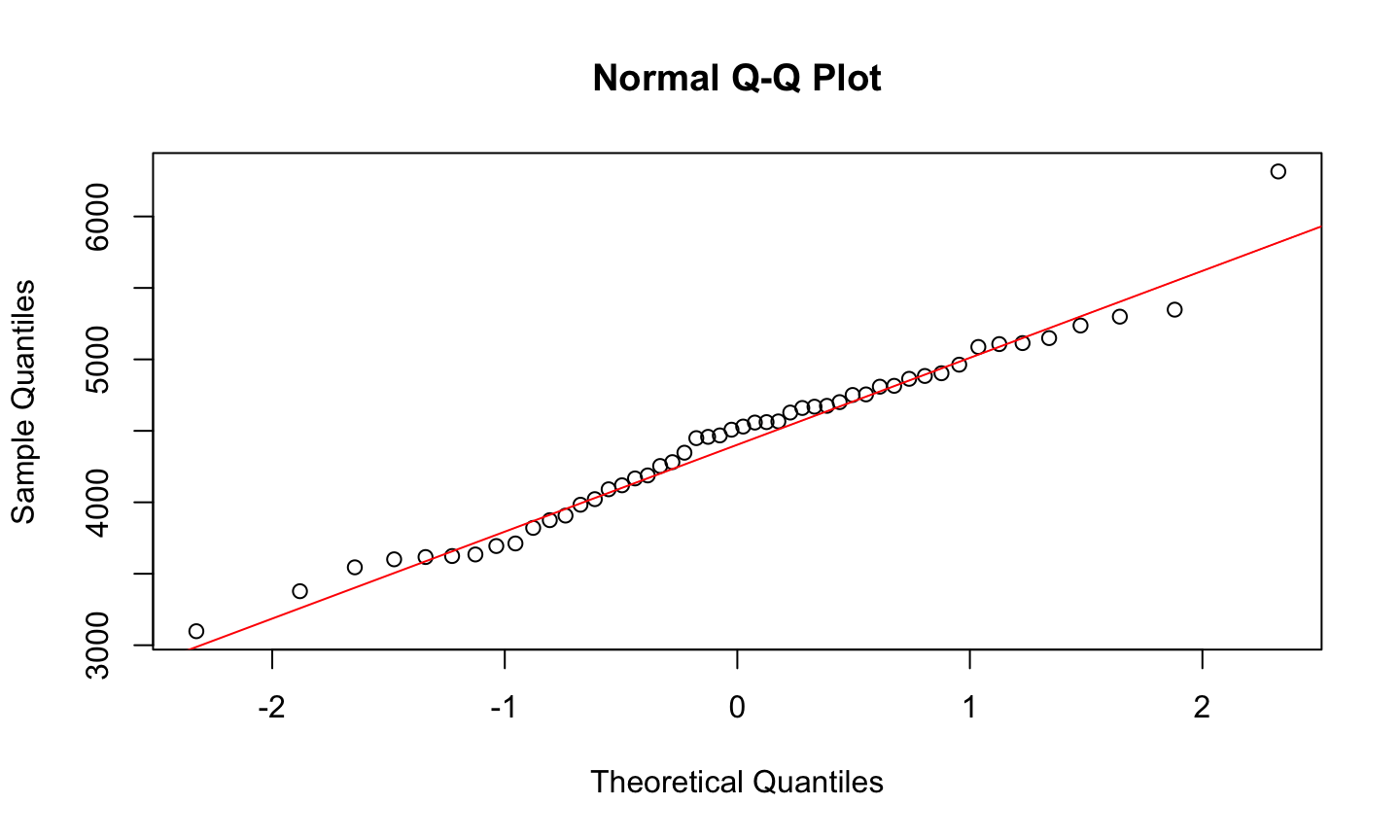## `qqnorm()` and `qqplot()`

```qqnorm(y)                             # qq plot for the earthquake depths
qqline(y, col = "red")                # red reference line```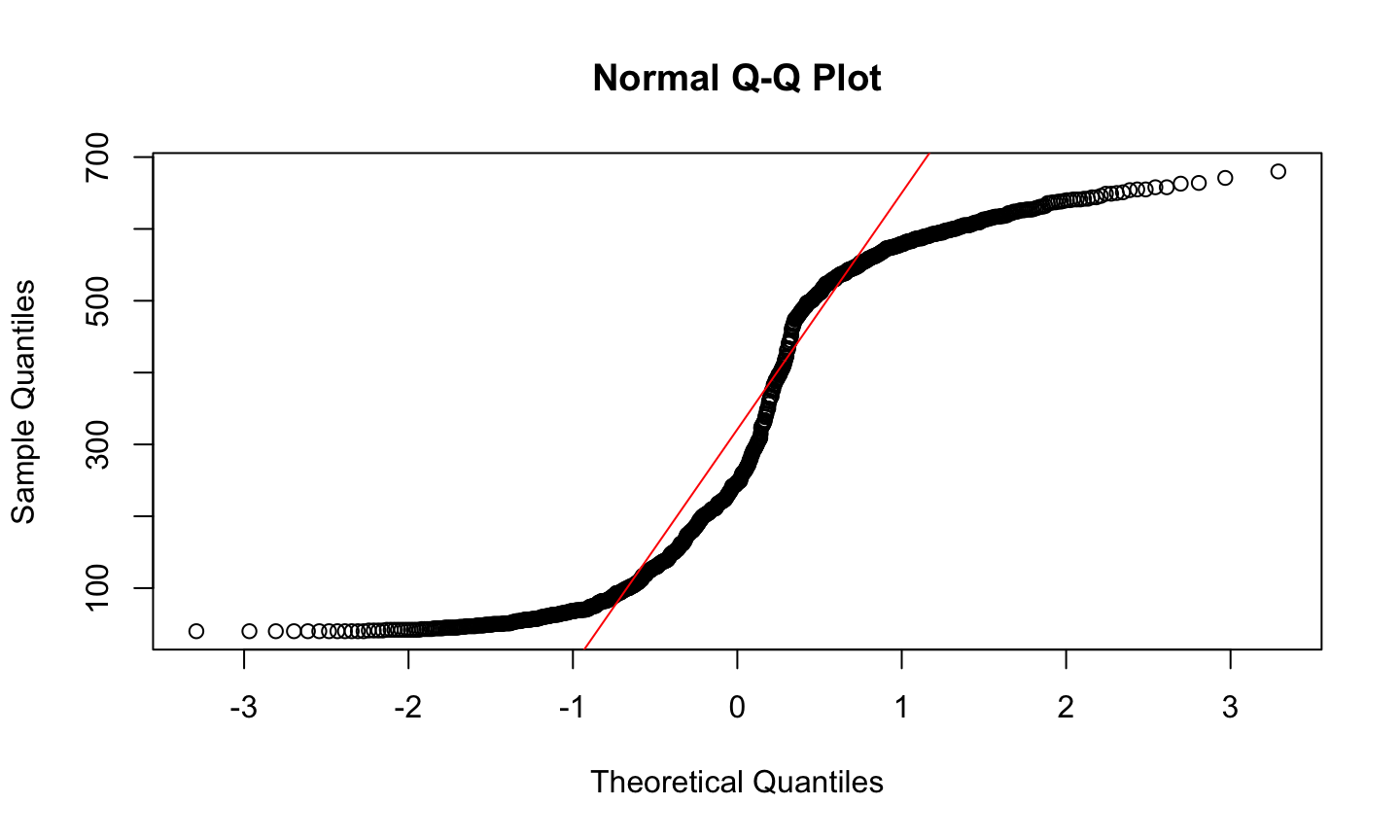## Box plots

`boxplot(count ~ spray, data = InsectSprays)`